# Test: Application Of Derivatives (CBSE Level) - 1

## 25 Questions MCQ Test Mathematics (Maths) Class 12 | Test: Application Of Derivatives (CBSE Level) - 1

Description
This mock test of Test: Application Of Derivatives (CBSE Level) - 1 for JEE helps you for every JEE entrance exam. This contains 25 Multiple Choice Questions for JEE Test: Application Of Derivatives (CBSE Level) - 1 (mcq) to study with solutions a complete question bank. The solved questions answers in this Test: Application Of Derivatives (CBSE Level) - 1 quiz give you a good mix of easy questions and tough questions. JEE students definitely take this Test: Application Of Derivatives (CBSE Level) - 1 exercise for a better result in the exam. You can find other Test: Application Of Derivatives (CBSE Level) - 1 extra questions, long questions & short questions for JEE on EduRev as well by searching above.
QUESTION: 1

### The instantaneous rate of change at t = 1 for the function f (t) = te−t + 9 is

Solution: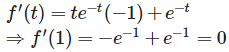QUESTION: 2

###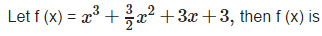Solution: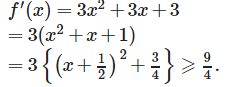QUESTION: 3

### The equation of the tangent to the curve y = e2x at the point (0, 1) is

Solution: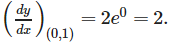Hence equation of tangent to the given curve at (0 , 1) is :

(y−1) = 2(x−0),i.e..y−1 = 2x

QUESTION: 4

The smallest value of the polynomial x3−18x2+96 in the interval [0, 9] is

Solution:

x3 - 18x2 + 96x = x(x2 - 18x + 96) = x[(x-9)2 + 15]
=x(x-9)+15 ≥ 0

QUESTION: 5

Let f (x) = (x2−4)1/3, then f has a

Solution: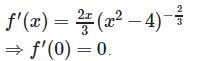Also, for x < 0 (slightly) , f ‘(x) < 0 and for x > 0 (slightly) f ‘(x) >0. Hence f has a local minima at x = 0 .

QUESTION: 6

If the graph of a differentiable function y = f (x) meets the lines y = – 1 and y = 1, then the graph

Solution:

Since the graph cuts the lines y = -1 and y = 1 , therefore ,it must cut y = 0 atleast once as the graph is a continuous curve in this case.

QUESTION: 7

Let f(x)  =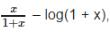where x>0, then f is

Solution: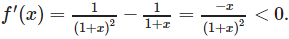QUESTION: 8

The equation of the tangent to the curve y=(4−x2)2/3 at x = 2 is

Solution: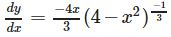, which does not exist at x = 2 . However , we find that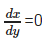, at x = 2 . Hence , there is a vertical tangent to the given curve at x = 2 .The point on the curve corresponding to x = 2 is (2 , 0). Hence , the equation of the tangent at x = 2 is x = 2

QUESTION: 9

Given that f (x) = x1/x , x>0, has the maximum value at x = e,then

Solution: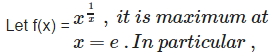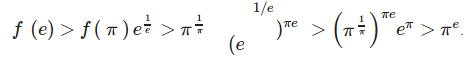QUESTION: 10

The function f (x) = x3 has a

Solution:

f ‘(0) = 0 , f ‘’ (0) = 0 and f ‘’’(0) = 6 . So, f has a point of inflexion at 0.

QUESTION: 11

Let f be a real valued function defined on (0, 1) ∪ (2, 4) such that f ‘ (x) = 0 for every x, then

Solution:

f ‘ (x) = 0 ⇒ f (x)is constant in (0 , 1)and also in (2, 4). But this does not mean that f (x) has the same value in both the intervals . However , if f (c) = f (d) , where c ∈ (0 , 1) and d ∈ (2, 4) then f (x) assumes the same value at all x ∈ (0 ,1) U (2, 4) and hence f is a constant function.

QUESTION: 12

Let f (x) = x4 – 4x, then

Solution:QUESTION: 13

The slope of the tangent to the curve x = a sin t, y = a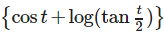at the point ‘t’ is

Solution: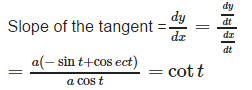QUESTION: 14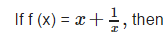Solution: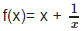has a local maximum value = - 2 at x = - 1 and a local minimum value = 2 at x = 1.

QUESTION: 15

The minimum value of (x) = sin x cos x is

Solution:

Sinx cosx = 1/2 (sin2x) and minimum value of sin2x is – 1 .

QUESTION: 16

In case of strict decreasing functions, slope of tangent and hence derivative is

Solution:

In case of a strict decreasing function , slope of tangent and hence derivative is either negative or zero.

QUESTION: 17

Let f (x) = x3−6x2+9x+18, then f (x) is strict decreasing in

Solution: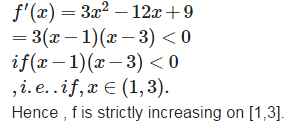QUESTION: 18

Tangents to the curve y = x3 at the points (1, 1) and (– 1, – 1) are

Solution: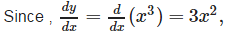therefore , slopes of the tangents at (1,1) and (- 1 , -1)are equal. Hence, the two tangents in reference are parallel.

QUESTION: 19

Let f(x) = x25(1−x)75 for all x ∈ [0,1], then f (x) assumes its maximum value at

Solution: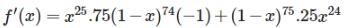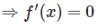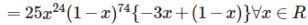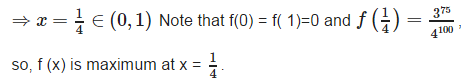QUESTION: 20

The stone projected vertically upwards moves under the action of gravity alone and its motion is described by x = 49 t – 4.9 t2 . It is at a maximum height when

Solution: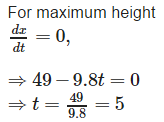QUESTION: 21

The function f (x) = 2 – 3 x is

Solution:

f (x) = 2 – 3x ⇒ f ‘ (x) = - 3 < 0 for all x ∈ R . so, f is strictly decreasing function.

QUESTION: 22

Let g (x) be continuous in a neighbourhood of ‘a’ and g (a) ≠ 0. Let f be a function such that f ‘ (x) = g(x) (x−a)2 , then

Solution:

Since g is continuous at a , therefore , if g (a) > 0 , then there is a nhd.of a, say (a-e , a+ e) in which g (x) is positive .This means that f ‘ (x)>0 in this nhd of a and hence f (x) is increasing at a.

QUESTION: 23

The line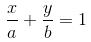touches the curvey =be-x/a at the point

Solution:

None of the given points in (b),(c) and (d) lies on the curve y = be−x/a.

QUESTION: 24

Minimum value of the function f(x) = x2+x+1 is

Solution: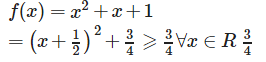QUESTION: 25

The function f (x) = | x | has

Solution:

The modulus function has V-shaped graph,which means that it has only one minima.